# Martin Orr's Blog

Maths > Abelian varieties > Absolute Hodge classes

## Absolute Hodge classes

Posted by Martin Orr on Thursday, 20 November 2014 at 18:55

Let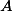be an abelian variety over a fieldof characteristic zero. For each embedding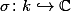, we get a complex abelian varietyby applyingto the coefficients of equations defining.

Whenever an object attached tois defined algebraically, we will get closely related objects for each. On the other hand, whenever we use complex analysis to define an object attached to, we should expect to get completely unrelated things for different(ifthen most field embeddings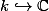are horribly discontinuous so will mess up anything analytic).

Hodge classes provide a special case: the definition of Hodge classes onasis analytic so we expect no relation between Hodge classes on different. But the Hodge conjecture says that every Hodge class in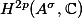is an algebraic cycle class, and this implies the associated cohomology class inis also a Hodge class for every. (We will explain in the post why there is a natural semilinear isomorphism.)

Deligne had the idea that we could pick this out as a partial step on the way to the Hodge conjecture: he defined an absolute Hodge class to be a cohomology class such that its associated class onis a Hodge class for everyand proved that every Hodge class on an abelian variety is an absolute Hodge class. It turns out that this is sufficient to obtain some of the consequences which would follow from the Hodge conjecture. In this post we will explain the definition of absolute Hodge classes.

### De Rham cohomology

So far in this blog, we have only seen an analytic construction of the cohomology with complex coefficientsof a complex abelian variety. Before we can talk about absolute Hodge classes, we need to construct this algebraically, so that we get semilinear isomorphisms betweenfor different, and hence know what it means to talk about a cohomology class being a Hodge class with respect to all.

Recall that a few posts ago, we constructed the tangent space of the universal vector extension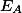. This is a vector space over. We proved that, looking at the complex abelian variety, there is a canonical isomorphismUsing the fact that the construction of the universal vector extension is compatible with extensions of the base field, for eachwe get a canonical isomorphismFollowing the fact that the cohomology groups ofare exterior powers of the dual of, we define the-th de Rham cohomology ofto be the-vector space(usually one would define de Rham cohomology in a more general way, for all algebraic varieties, and then it is a theorem that the above formula holds for abelian varieties). We get canonical isomorphisms### De Rham cohomology and the Hodge filtration

As we discussed previously for, the Hodge decomposition forcannot defined algebraically (it cannot come from a decomposition of the-vector space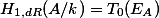) but the Hodge filtration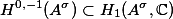corresponds to the first part of the exact sequence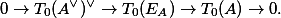Dualising, we get thatmaps the filtrationtoWe can define a descending filtration on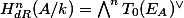by lettingbe the subspace generated by elements of the form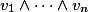whereand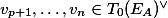. Then the above gives usor in other words "the Hodge filtration is defined algebraically."

### Hodge classes and de Rham cohomology

We say that a de Rham cohomology classis a Hodge class relative toif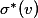is a Hodge class oni.e. if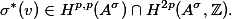We can equivalently saybecause ifthenis its own complex conjugate (because it is in) and so the complex conjugation condition on the Hodge decomposition forces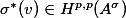.

Observe that the conditionis independent of, while the conditiondepends onand becauseis an analytic object, even if the second condition holds for one, it probably does not hold for most.

### Enter the Hodge conjecture

Suppose that we have a cohomology classwhich is a Hodge class relative to one embedding. The Hodge conjecture says thatis in the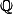-span of the classes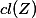of algebraic subvarieties.

Now suppose we are given another embedding, and suppose that there is an automorphismof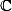such that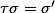. (Such adoes not always exist whenbecause there are non-surjective embeddings. There is a way of dealing withfor which there is no, but we will ignore them for now.)

Then applyingto the coefficients of equations defining the subvariety, we get a subvariety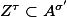. Furthermore, there is an algebraic recipe for defining cycle classes in de Rham cohomology, and this implies thatHenceis an algebraic cycle class, and hence a Hodge class.

Thus the Hodge conjecture implies thatis a Hodge class for every.

We define an absolute Hodge class to be a de Rham cohomology classwhich is a Hodge class relative to every. Deligne proved:

Theorem. Ifis an abelian variety overandis a Hodge class relative to one embedding, thenis an absolute Hodge class.

By the above argument, the Hodge conjecture would imply that the theorem is true for all algebraic varieties. However it is only known for abelian varieties, K3 surfaces and a few other special cases.

1. Deligne's theorem on absolute Hodge classes From Martin's Blog

Today I will outline the proof of Deligne's theorem that Hodge classes on an abelian variety are absolute Hodge. The proof goes through three steps of reducing to increasingly special types of abelian varieties, until finally one reaches a case ...

2. Deligne's Principle B From Martin's Blog

As I explained last time, one of the key steps in the the proof of Deligne's theorem on absolute Hodge classes is Principle B. This allows us to take an absolute Hodge classes on one fibre in a family of varieties, and deduce that certain classes...

3. Tate twists in singular and de Rham cohomology From Martin's Blog

Tate twists in singular cohomology are a device for dealing with factors of 2 pi i which come up whenever we compare singular and de Rham cohomology of complex projective varieties. In this post I will explain the problem, including calculating...

4. Absolute Hodge classes in l-adic cohomology From Martin's Blog

We can define absolute Hodge classes in l-adic cohomology in the same way as absolute Hodge classes in de Rham cohomology. We can then prove Deligne's theorem, that Hodge classes on an abelian variety are absolute Hodge, for l-adic cohomology. ...

5. Motivic Galois groups and periods From Martin's Blog

In my last post, I discussed ... an upper bound for the transcendence degree of the extended period matrix of an abelian variety, namely the dimension of the general symplectic group. ... In this post, I will discuss how this can be generalised ...